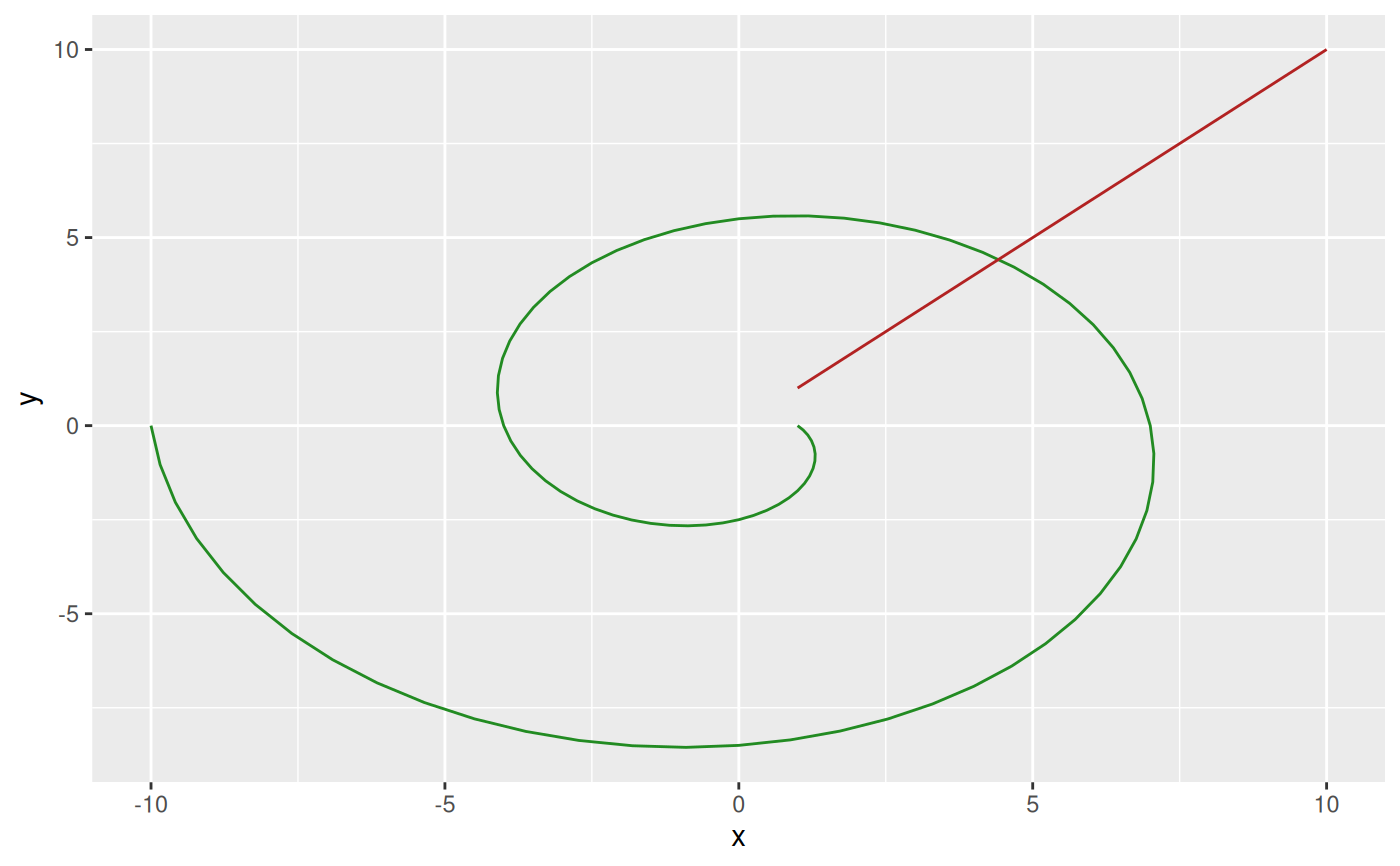This function creates a trans object that converts radial data to their corresponding coordinates in cartesian space. The trans object is created for a specific radius and angle range that will be mapped to the unit circle so data doesn't have to be normalized to 0-1 and 0-2*pi in advance. While there exists a clear mapping from radial to cartesian, the inverse is not true as radial representation is periodic. It is impossible to know how many revolutions around the unit circle a point has taken from reading its coordinates. The inverse function will always assume that coordinates are in their first revolution i.e. map them back within the range of a.range.

radial_trans(r.range, a.range, offset = pi/2, pad = 0.5, clip = FALSE)

## Arguments

r.range

The range in radius that correspond to 0 - 1 in the unit circle.

a.range

The range in angles that correspond to 2*pi - 0. As radians are normally measured counterclockwise while radial displays are read clockwise it's an inverse mapping

offset

The offset in angles to apply. Determines that start position on the circle. pi/2 (the default) corresponds to 12 o'clock.

Adds to the end points of the angle range in order to separate the start and end point. Defaults to 0.5

clip

Should input data be clipped to r.range and a.range or be allowed to extend beyond. Defaults to FALSE (no clipping)

## Value

A trans object. The transform method for the object takes an r (radius) and a (angle) argument and returns a data.frame with x and y columns with rows for each element in r/a. The inverse method takes an x and y argument and returns a data.frame with r and a columns and rows for each element in x/y.

## Note

While trans objects are often used to modify scales in ggplot2, radial transformation is different as it is a coordinate transformation and takes two arguments. Consider it a trans version of coord_polar and use it to transform your data prior to plotting.

## Examples

# Some data in radial form
rad <- data.frame(r = seq(1, 10, by = 0.1), a = seq(1, 10, by = 0.1))

# Create a transformation
cart <- radial$transform(rad$r, rad\$a)